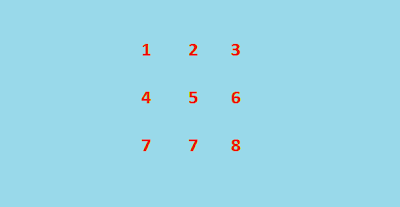## Thursday, 20 December 2012

### Gauss Jordan Elimination: C/C++ Program

Gauss Jordan elimination is one of the many operations we can perform on matrices. Here I have provided a program which finds the diagonal matrix corresponding to the given matrix. It is often used to solve system of linear equations. Elementary row operations are used to obtain the diagonal matrix in Gauss Jordan elimination method.A 3 X 3 Matrix
The Figure Below Shows the Diagonal Matrix Obtained by Gauss Jordan Elimination.